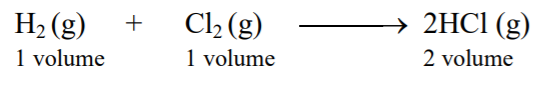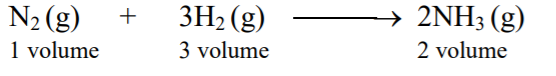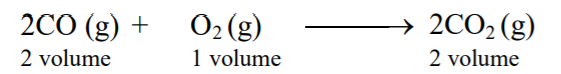# Gay Lussac's law of Gaseous Volume↪ This law was stated by Gay-Lussac in 1805 A.D.
↪ This law is applied only for gaseous reactions i.e. in which all the reactants and products are gases.
↪ It states that, ''when gaseous reactants react to give gaseous products, they all bear simple volume ratio under similar condition of temperature and pressure.'' This law can be explained by considering different gaseous reactions. For example,Above reaction is a gaseous reaction. Under similar condition of temperature and pressure, the volume ratio of reactants and products is 1 : 1 : 2. This is a simple whole number ratio, which satisfies the law of gaseous volume.Under similar condition of temperature and pressure, the volume ratio of reactants and products is 1 : 3 : 2, which is simple whole number ratio. So, this example also explains law of gaseous volume.Volume ratio of reactants and products is 2 : 1 : 2, which is again simple whole number ratio. Hence, above gaseous reaction illustrates law of gaseous volume.